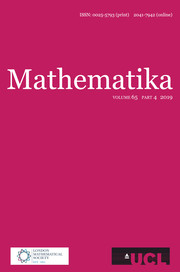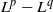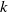Home
Hostname: page-component-5c569c448b-ckh7h Total loading time: 0.795 Render date: 2022-07-06T07:05:58.640Z Has data issue: true Feature Flags: { "shouldUseShareProductTool": true, "shouldUseHypothesis": true, "isUnsiloEnabled": true, "useRatesEcommerce": false, "useNewApi": true } hasContentIssue trueMathematika

# AN AFFINE FOURIER RESTRICTION THEOREM FOR CONICAL SURFACES

Published online by Cambridge University Press:  13 December 2013

## Abstract

A Fourier restriction estimate is obtained for a broad class of conic surfaces by adding a weight to the usual underlying measure. The new restriction estimate exhibits a certain affine-invariance and implies the sharp${L}^{p} - {L}^{q}$ restriction theorem for compact subsets of a type$k$ conical surface, up to an endpoint. Furthermore, the chosen weight is shown to be, in some quantitative sense, optimal. Appended is a discussion of type$k$ conical restriction theorems which addresses some anomalies present in the existing literature.

## MSC classification

Type
Research Article
Information
Mathematika , July 2014 , pp. 374 - 390

## Access options

Get access to the full version of this content by using one of the access options below. (Log in options will check for institutional or personal access. Content may require purchase if you do not have access.)

## References

Abi-Khuzam, F. and Shayya, B., Fourier restriction to convex surfaces of revolution in${ \mathbb{R} }^{3}$. Publ. Mat. 50 (1) (2006), 7185.CrossRefGoogle Scholar
Bak, J.-G. and Seeger, A., Extensions of the Stein–Tomas theorem. Math. Res. Lett. 18 (4) (2011), 767781.CrossRefGoogle Scholar
Barceló, B., On the restriction of the Fourier transform to a conical surface. Trans. Amer. Math. Soc. 292 (1) (1985), 321333.Google Scholar
Barceló, B., The restriction of the Fourier transform to some curves and surfaces. Studia Math. 84 (1) (1986), 3969.CrossRefGoogle Scholar
Buschenhenke, S., A sharp${L}_{p} - {L}_{q}$ Fourier restriction theorem for a conical surface of finite type, Preprint, 2012, arXiv:1208.5876v1 [math.CA].Google Scholar
Carbery, A., Christ, M. and Wright, J., Multidimensional van der Corput and sublevel set estimates. J. Amer. Math. Soc. 12 (4) (1999), 9811015.Google Scholar
Carbery, A., Kenig, C. and Ziesler, S., Restriction for flat surfaces of revolution in${\mathbf{R} }^{3}$. Proc. Amer. Math. Soc. 135 (6) (2007), 19051914; electronic.CrossRefGoogle Scholar
Carbery, A. and Ziesler, S., Restriction and decay for flat hypersurfaces. Publ. Mat. 46 (2) (2002), 405434.CrossRefGoogle Scholar
Drury, S. W., Degenerate curves and harmonic analysis. Math. Proc. Cambridge Philos. Soc. 108 (1) (1990), 8996.CrossRefGoogle Scholar
Drury, S. W. and Guo, K., Some remarks on the restriction of the Fourier transform to surfaces. Math. Proc. Cambridge Philos. Soc. 113 (1) (1993), 153159.CrossRefGoogle Scholar
Federer, H., Geometric Measure Theory (Classics in Mathematics), Springer (1996).CrossRefGoogle Scholar
Iosevich, A. and Lu, G., Sharpness results and Knapp’s homogeneity argument. Canad. Math. Bull. 43 (1) (2000), 6368.CrossRefGoogle Scholar
Nicola, F., A note on the restriction theorem and geometry of hypersurfaces. Math. Scand. 103 (1) (2008), 5360.CrossRefGoogle Scholar
Nicola, F., Slicing surfaces and the Fourier restriction conjecture. Proc. Edinb. Math. Soc. (2) 52 (2) (2009), 515527.CrossRefGoogle Scholar
Oberlin, D. M., Fourier restriction for affine arclength measures in the plane. Proc. Amer. Math. Soc. 129 (11) (2001), 33033305; electronic.CrossRefGoogle Scholar
Oberlin, D. M., Some convolution inequalities and their applications. Trans. Amer. Math. Soc. 354 (6) (2002), 25412556; electronic.CrossRefGoogle Scholar
Oberlin, D. M., A uniform Fourier restriction theorem for surfaces in${ \mathbb{R} }^{3}$. Proc. Amer. Math. Soc. 132 (4) (2004), 11951199; electronic.CrossRefGoogle Scholar
Oberlin, D. M., A uniform Fourier restriction theorem for surfaces in${ \mathbb{R} }^{d}$. Proc. Amer. Math. Soc. 140 (1) (2012), 263265.CrossRefGoogle Scholar
Shayya, B., An affine restriction estimate in${ \mathbb{R} }^{3}$. Proc. Amer. Math. Soc. 135 (4) (2007), 11071113; electronic.CrossRefGoogle Scholar
Shayya, B., Affine restriction for radial surfaces. Math. Z. 262 (1) (2009), 4155.CrossRefGoogle Scholar
Sjölin, P., Fourier multipliers and estimates of the Fourier transform of measures carried by smooth curves in${R}^{2}$. Studia Math. 51 (1974), 169182.CrossRefGoogle Scholar
Sogge, C. D., A sharp restriction theorem for degenerate curves in${\mathbf{R} }^{2}$. Amer. J. Math. 109 (2) (1987), 223228.CrossRefGoogle Scholar
Stein, E. M., Harmonic Analysis: Real-Variable Methods, Orthogonality, and Oscillatory Integrals (Princeton Mathematical Series 43), Princeton University Press (1993).Google Scholar
Tao, T., Some recent progress on the restriction conjecture. In Fourier Analysis and Convexity (Applied and Numerical Harmonic Analysis), Birkhäuser (Boston, MA, 2004), 217243.CrossRefGoogle Scholar
1
Cited by

# Save article to Kindle

Note you can select to save to either the @free.kindle.com or @kindle.com variations. ‘@free.kindle.com’ emails are free but can only be saved to your device when it is connected to wi-fi. ‘@kindle.com’ emails can be delivered even when you are not connected to wi-fi, but note that service fees apply.

Find out more about the Kindle Personal Document Service.

AN AFFINE FOURIER RESTRICTION THEOREM FOR CONICAL SURFACES
Available formats
×

# Save article to Dropbox

To save this article to your Dropbox account, please select one or more formats and confirm that you agree to abide by our usage policies. If this is the first time you used this feature, you will be asked to authorise Cambridge Core to connect with your Dropbox account. Find out more about saving content to Dropbox.

AN AFFINE FOURIER RESTRICTION THEOREM FOR CONICAL SURFACES
Available formats
×

# Save article to Google Drive

To save this article to your Google Drive account, please select one or more formats and confirm that you agree to abide by our usage policies. If this is the first time you used this feature, you will be asked to authorise Cambridge Core to connect with your Google Drive account. Find out more about saving content to Google Drive.

AN AFFINE FOURIER RESTRICTION THEOREM FOR CONICAL SURFACES
Available formats
×
×